# OR-Notes

## J E Beasley

OR-Notes are a series of introductory notes on topics that fall under the broad heading of the field of operations research (OR). They were originally used by me in an introductory OR course I give at Imperial College. They are now available for use by any students and teachers interested in OR subject to the following conditions.

A full list of the topics available in OR-Notes can be found here.

#### Network analysis example 1990 UG exam

The table below defines the activities within a small project.

```Activity   Start   End    Completion time
node    node   (weeks)
1          1       2       2
2          1       3       3
3          1       4       2
4          2       5       3
5          3       6       7
6          4       6       5
7          5       7       4
8          6       7       9
9          7       8       3 ```

In addition to the above information we have that activity 7 cannot start until activity 5 has been completed.

• Draw the network diagram.
• Calculate the minimum overall project completion time.
• Calculate the float time for each activity and hence identify the activities which are critical.

#### Solution

The network diagram is shown below. Note the introduction of node 9 and dummy activities 10 and 11 to correctly represent the problem as described in the question.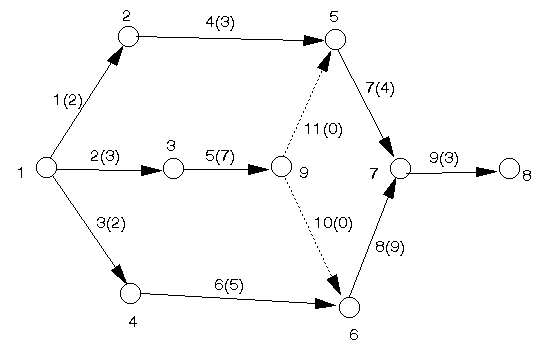We then have the following calculation of earliest start times to determine the minimum overall project completion time.

E1 = 0 (by definition)
E2 = E1 + T12 = 0 + 2 = 2
E3 = E1 + T13 = 0 + 3 = 3
E4 = E1 + T14 = 0 + 2 = 2
E5 = max[E2 + T25, E9 + T95]

but we have not yet worked out E9 so we must leave this calculation until we know E9.

Similarly it can be seen that we cannot yet work out E6, E7 and E8 until we calculate E9.

E9 = E3 + T39 = 3 + 7 = 10

We can now calculate E5 since we now know E9.

E5 = max[E2 + T25, E9 + T95] = max[2 + 3, 10 + 0] = 10
E6 = max[E4 + T46, E9 + T96] = max[2 + 5, 10 + 0] = 10
E7 = max[E5 + T57, E6 + T67] = max[10 + 4, 10 + 9] = 19
E8 = E7 + T78 = 19 + 3 = 22

Hence the minimum overall project completion time is 22 weeks.

To determine the float times and which activities are critical we need to work out the latest start times.

L8 = 22 (by definition)
L7 = L8 - T78 = 22 - 3 = 19
L6 = L7 - T67 = 19 - 9 = 10
L5 = L7 - T57 = 19 - 4 = 15
L9 = min[L5 - T95, L6 - T96] = min[15 - 0, 10 - 0] = 10
L4 = L6 - T46 = 10 - 5 = 5
L3 = L9 - T39 = 10 - 7 = 3
L2 = L5 - T25 = 15 - 3 = 12
L1 = min[L2 - T12, L3 - T13, L4 - T14] = min[12-2, 3-3, 5-2] = 0

and note that L1 = 0 as required.

To calculate the float times we use the equation Fij = Lj - Ei - Tij to get

```Activity i   j  Lj    Ei    Tij    Fij
1        1   2  12    0     2     10
2        1   3  3     0     3     0
3        1   4  5     0     2     3
4        2   5  15    2     3     10
5        3   9  10    3     7     0
6        4   6  10    2     5     3
7        5   7  19    10    4     5
8        6   7  19    10    9     0
9        7   8  22    19    3     0
10       9   6  10    10    0     0
11       9   5  15    10    0     5
```

Note here that all float times are >= 0 as required. Hence the critical activities (those with a float of zero) are 2,5,8,9 and 10 and these form the critical path from the start node (node 1) to the finish node (node 8) in the network.

#### Network analysis example 1989 UG exam

The table below defines the activities within a small project.

```Activity   Start   End    Completion time
node    node   (weeks)
1          1       2      2
2          1       3      3
3          3       4      7
4          3       5      6
5          2       7      10
6          4       5      1
7          5       6      3
8          6       7      4
9          4       7      7
10         7       8      3
```

In addition to the above information we have that activity 7 cannot start until activity 1 has been completed and activity 10 cannot start until activity 6 has been completed.

• Draw the network diagram.
• Calculate the minimum overall project completion time.
• Calculate the float time for each activity and hence identify the activities which are critical.

#### Solution

The network diagram is shown below.

Note the introduction of node 9 and dummy activities 11, 12 and 13 to correctly represent the problem as described in the question.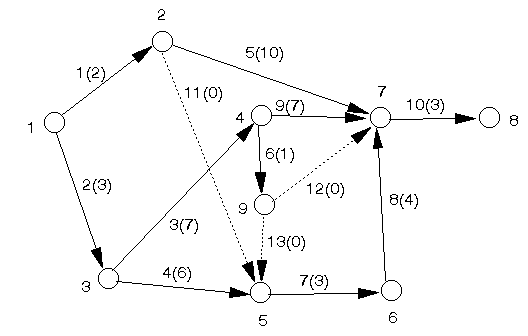We then have the following calculation of earliest start times to determine the minimum overall project completion time.

E1 = 0 (by definition)
E2 = E1 + T12 = 0 + 2 = 2
E3 = E1 + T13 = 0 + 3 = 3
E4 = E3 + T37 = 3 + 7 = 10
E5 = max[E2 + T25, E3 + T35, E9 + T95]

but we have not yet worked out E9 so we must leave this calculation until we know E9.

In fact it can be seen that, for similar reasons, we cannot yet calculate E6, E7 and E8 so we next calculate E9.

E9 = E4 + T49 = 10 + 1 = 11

We can now calculate E5 since we now know E9

E5 = max[E2 + T25, E3 + T35, E9 + T95] = max[2+0, 3+6, 11+0] = 11
E6 = E5 + T56 = 11 + 3 = 14
E7 = max[E2 + T27, E4 + T47, E6 + T67, E9 + T97] = max[2+10, 10+7, 14+4, 11+0] = 18
E8 = E7 + T78 = 18 + 3 = 21

Hence the minimum overall project completion time is 21 weeks.

To determine the float times and which activities are critical we need to work out the latest start times.

L8 = 21 (by definition)
L7 = L8 - T78 = 21 - 3 = 18
L6 = L7 - T67 = 18 - 4 = 14
L5 = L6 - T56 = 14 - 3 = 11
L4 = min[L7 - T47, L9 - T49]

but we have not yet worked out L9 and so we must leave the calculation until we know L9

L9 = min[L7 - T97, L5 - T95] = min[18 - 0, 11 - 0] = 11

We can now calculate L4 since we now know L9

L4 = min[L7 - T47, L9 - T49] = min[18 - 7, 11 - 1] = 10
L3 = min[L4 - T34, L5 - T35] = min[10 - 7, 11 - 6] = 3
L2 = min[L7 - T27, L5 - T25] = min[18 - 10, 11 - 0] = 8
L1 = min[L3 - T13, L2 - T12] = min[3 - 3, 8 - 2] = 0

and note that L1 = 0 as required.

To calculate the float times we use the equation Fij = Lj - Ei - Tij to get

```Activity i   j  Lj    Ei    Tij    Fij
1        1   2  8     0     2     6
2        1   3  3     0     3     0
3        3   4  10    3     7     0
4        3   5  11    3     6     2
5        2   7  18    2     10    6
6        4   9  11    10    1     0
7        5   6  14    11    3     0
8        6   7  18    14    4     0
9        4   7  18    10    7     1
10       7   8  21    18    3     0
11       2   5  11    2     0     9
12       9   7  18    11    0     7
13       9   5  11    11    0     0
```

Note here that all float times are >= 0 as required.

Hence the critical activities (those with a float of zero) are 2,3,6,7,8,10 and 13 and these form the critical path from the start node (node 1) to the finish node (node 8) in the network.

#### Network analysis example 1988 UG exam

The table below defines the various activities within a small project.

```Activity   Start   End    Completion time
node    node   (weeks)
1          1       3      1
2          2       4      5
3          1       2      2
4          2       3      4
5          4       7      7
6          4       5      2
7          5       6      2
8          6       7      3
9          7       8      2
10         4       8      6
11         3       6      5
```

In addition to the above information we have that activity 11 cannot start until activity 2 has been completed.

• Draw the network diagram.
• Calculate the minimum overall project completion time.
• Which activities are critical?

Comment on the progress of the project, and the chances of achieving the minimum overall project completion time as calculated above, if at the end of 11 weeks, the status of the activities is as follows:

• Finished - 1,2,3,4,6
• Under way - 5 (3 weeks to completion), 10 (2 weeks to completion), 11 (2 weeks to completion)

Formulate the problem of determining the minimum overall project completion time for the project defined above as a linear program. Explain why your linear program is a correct mathematical description of the problem.

#### Solution

The network diagram is shown below

Note the use of the dummy activity (activity 12) between nodes 4 and 3 to represent the condition that activity 11 cannot start until activity 2 has been completed.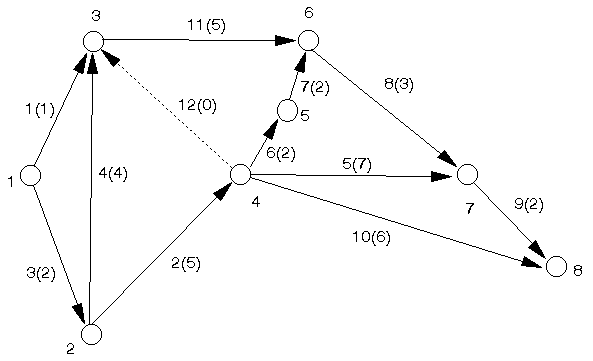We then have the following calculation of earliest start times to determine the minimum overall project completion time.

E1 = 0 (by definition)
E2 = E1 + T12 = 0 + 2 = 2
E3 = max[E1 + T13, E2 + T23, E4 + T43]

but we have not yet worked out E4 so we must leave this calculation until we know E4

E4 = E2 + T24 = 2 + 5 = 7

We can now calculate E3 since we now know E4

E3 = max[E1 + T13, E2 + T23, E4 + T43] = max[0+1, 2+4, 7+0] = 7
E5 = E4 + T45 = 7 + 2 = 9
E6 = max[E5 + T56, E3 + T36] = max[9 + 2, 7 + 5] = 12
E7 = max[E6 + T67, E4 + T47] = max[12 + 3, 7 + 7] = 15
E8 = max[E7 + T78, E4 + T48] = max[15 + 2, 7 + 6] = 17

Hence the minimum overall project completion time is 17 weeks.

To determine which activities are critical we need to work out the latest start times and then the float times.

L8 = 17 (by definition)
L7 = L8 - T78 = 17 - 2 = 15
L6 = L7 - T67 = 15 - 3 = 12
L5 = L6 - T56 = 12 - 2 = 10
L4 = min[L5 - T45, L7 - T47, L8 - T48, L3 - T43]

but we have not yet worked out L3 so we must leave this calculation until we know L3

L3 = L6 - T36 = 12 - 5 = 7

We can now calculate L4 since we now know L3

L4 =min[L5 - T45, L7 - T47, L8 - T48, L3 - T43] = min[10 - 2, 15 - 7, 17 - 6, 7 - 0] = 7
L2 = min[L3 - T23, L4 - T24] = min[7 - 4, 7 - 5] = 2
L1 = min[L3 - T13, L2 - T12] = min[7 - 1, 2 - 2] = 0

and note that L1 = 0 as required.

To calculate the float times we use the equation Fij=Lj-Ei-Tij to get the table below

```Activity i   j  Lj    Ei    Tij    Fij
1        1   3  7     0    1      6
2        2   4  7     2    5      0
3        1   2  2     0    2      0
4        2   3  7     2    4      1
5        4   7  15    7    7      1
6        4   5  10    7    2      1
7        5   6  12    9    2      1
8        6   7  15    12   3      0
9        7   8  17    15   2      0
10       4   8  17    7    6      4
11       3   6  12    7    5      0
Dummy    4   3  7     7    0      0
```

Note here that all floats are >= 0 as required.

Hence the critical activities (those with a float of zero) are 2,3,8,9,11 and 12 and these form the critical path from the start node (node 1) to the finish node (node 8) in the network.

Given the information in the question on the status of the project we can revise the network diagram to that shown below where:

• we remove from the network all completed activities; and
• we redraw all activities currently under way as emanating from a new start node (and change their completion times accordingly). ]

Note here that as activity 11 is under way we can assume that activity 12 (the dummy activity linking the finish of activity 2 to the start of activity 11) has also been completed. Note too that activity 7 also emanates from node 1 as it is free to start.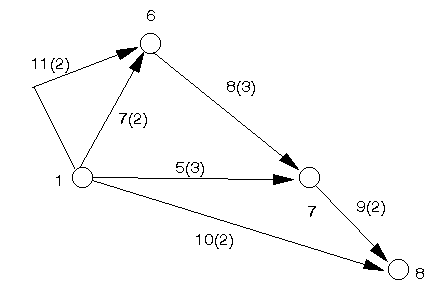We redraw this network since we have two arcs linking node 1 and node 6 and, by convention, no two nodes in a network ought to be directly connected by more than one arc. To overcome this we introduce an artificial node (node 2, say) and a dummy activity (activity 13, say) to get the diagram below.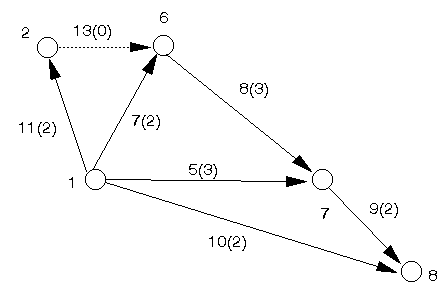We calculate earliest start times for the above network to determine the minimum overall completion time for the remaining activities.

E1 = 0 (by definition)
E2 = E1 + T12 = 0 + 2 = 2
E6 = max[E1 + T16, E2 + T26] = max[0 + 2, 2 + 0] = 2
E7 = max[E1 + T17, E6 + T67] = max[0 + 3, 2 + 3] = 5
E8 = max[E1 + T18, E7 + T78] = max[0 + 2, 5 + 2] = 7

Hence the minimum overall completion time for the remaining activities is 7 weeks.

As 11 weeks have already elapsed we will complete the entire project in 11 + 7 = 18 weeks and this compares with the completion time of 17 weeks we calculated above. Hence, at some stage, we have slipped a week and the project is currently a week late. There is therefore no chance of achieving the previous completion time of 17 weeks unlesss some activities can be speeded up (crashed)

As before, letting Ei represent the earliest start time for activity i we have the LP

minimise E8

subject to

E1 = 0
E2 = E1 + T12
E3 >= E1 + T13
E3 >= E2 + T23
E3 >= E4 + T43
E4 = E2 + T24
E5 = E4 + T45
E6 >= E5 + T56
E6 >= E3 + T36
E7 >= E6 + T67
E7 >= E4 + T47
E8 >= E7 + T78
E8 >= E4 + T48

Ei >= 0 i=1,2,...,8

This LP is a correct description of the problem as comparing it with the steps we carried out above to calculate E8 (the minimum overall project completion time) we have that:

• the objective is to minimise E8 (effectively as before)
• all equality relationships are as before

The change occurs with respect to max[.,.] terms, e.g. consider E6=max[E5+T56, E3+T36].

In the LP above this is regarded as equivalent to the two linear constraints

E6 >= E5 + T56
E6 >= E3 + T36

In the optimal solution to the LP given above we therefore have:

• if either (or both) of these two constraints is satisfied with equality then E6=max[E5+T56, E3+T36] is also satisfied
• if neither of these two constraints is satisfied with equality i.e. E6 > E5 + T56 and E6 > E3 + T36 then E6=max[E5+T56, E3+T36] will not be satisfied. Hence in this case the LP is producing an incorrect value for E6.

However the LP cannot produce an incorrect value for E8 (the objective) since we have the minimum value of E8 and if it were worthwhile reducing E6 to reduce E8 we would have done so.

This illustrates that although the LP produces a correct value for E8 (and hence is a correct description of the problem) it does not necessarily produce correct values for other Ei (1<=i<=7). For this reason we (strictly) need to change our definition of Ei for the LP to avoid the term "earliest". Hence we redefine Ei to be merely some time at which node i is reached (with all preceding activities completed).

#### Network analysis example 1986 UG exam

A project consists of 14 activities (1,2,3,...,14). The duration of each activity and the activities which must immediately precede it are shown below. Draw a network which represents these activities and their precedence relationships.

```Activity  Preceding  Duration
Activities (weeks)
1         -           1
2         1           3
3         2           2
4         2           2
5         2           4
6         4           1
7         3,6         4
8         6           2
9         6           1
10        3,5,8,9     8
11        7,10        2
12        11          2
13        7           4
14        12,13       2
```

The figure below shows a first attempt at solving the problem - it can be tidied up/improved e.g. by numbering the nodes.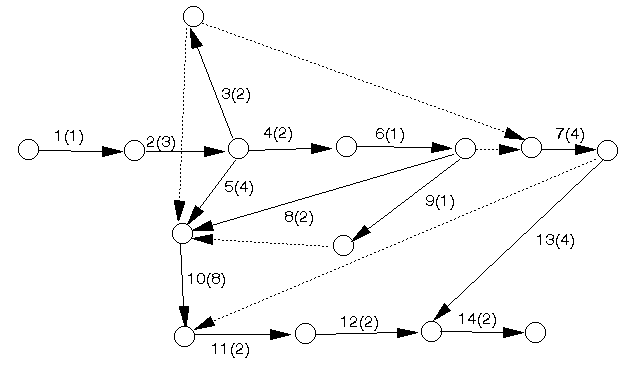#### Network analysis example

For the network shown below what are the critical activities?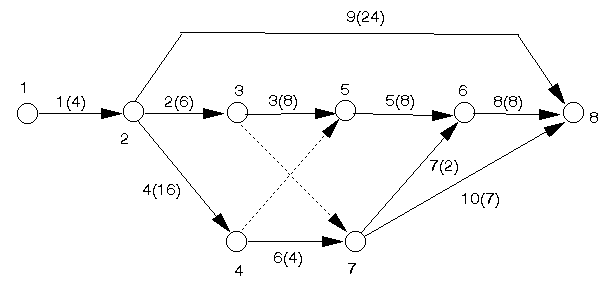#### Earliest start times

E1 = 0 (by definition)
E2 = E1 + T12 = 0 + 4 = 4
E3 = E2 + T23 = 4 + 6 = 10
E4 = E2 + T24 = 4 + 16 = 20
E5 = max[E3 + T35, E4 + T45] = max[10 + 8, 20 + 0] = 20
E6 = max[E5 + T56, E7 + T76]

but we have not yet worked out E7 so we must leave this calculation until we know E7 (this often happens and merely indicates that a different numbering of the nodes would have been more appropriate)

E7 = max[E3 + T37, E4 + T47] = max[10 + 0, 20 + 4] = 24

We can now calculate E6 since we now know E7

E6 = max[E5 + T56, E7 + T76] = max[20 + 8, 24 + 2] = 28
E8 = max[E2 + T28, E6 + T68, E7 + T78] = max[4 + 24, 28 + 8, 24 + 7] = 36

Hence the minimum completion time for the project is 36.

#### Latest start times

L8 = E8 = 36 (by definition)
L7 = min[L8 - T78, L6 - T76]

and we do not yet know L6 so we must leave this calculation until we do

L6 = L8 - T68 = 36 - 8 = 28

We can now calculate L7 since we now know L6

L7 = min[L8 - T78, L6 - T76] = min[36 - 7, 28 - 2] = 26
L5 = L6 - T56 = 28 - 8 = 20
L4 = min[L5 - T45, L7 - T47] = min[20 - 0, 26 - 4] = 20
L3 = min[L5 - T35, L7 - T37] = min[20 - 8, 26 - 0] = 12
L2 = min[L8 - T28, L3 - T23, L4 - T24] = min[36 - 24, 12 - 6, 20 - 16] = 4
L1 = L2 - T12 = 4 - 4 = 0 (as required)

#### Float

We have the table

```Activity i   j  Lj    Ei    Tij    Fij
1        1   2  4     0     4     0
2        2   3  12    4     6     2
3        3   5  20   10     8     2
4        2   4  20    4     16    0
5        5   6  28    20    8     0
6        4   7  26    20    4     2
7        7   6  28    24    2     2
8        6   8  36    28    8     0
9        2   8  36    4     24    8
10       7   8  36    24    7     5
Dummy    4   5  20    20    0     0
Dummy    3   7  26    10    0     16
```

Note that all floats are >= 0 as required.

Hence the critical activities are 1, 4, 5, 8 and these (together with the dummy activity between node 4 and node 5) form the critical path from the start node to the final node.

#### Network analysis example

The table below defines the various activities in a small project.

```Activity  Start node  End node  Completion time (weeks)
1         1           2         2
2         1           3         3
3         2           4         4
4         2           5         1
5         3           5         2
6         3           6         3
7         6           5         1
8         4           7         2
9         5           7         4
10        6           7         2
```

In addition activities 3 and 4 cannot start until activity 2 has been completed.

Draw the network diagram, calculate the earliest and latest start times and hence find the float for each activity, the critical activities and the overall project completion time.

How would the overall project completion time be affected by:

• an increase in the completion time for activity 1 of 2 weeks
• a decrease in the completion time for activity 10 of 1 week
• an increase in the completion time for activity 6 of 2 weeks.

#### Solution

The network diagram is shown below and note the dummy activity between nodes 3 and 2 to represent the condition that activities 3 and 4 cannot start until activity 2 has been completed.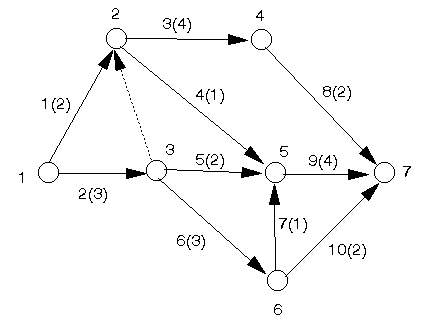We have the following calculations.

#### Earliest start times

E1 = 0 (by definition)
E2 = max[E1 + T12, E3 + T32]

but we have not yet worked out E3 so we leave this until we have calculated it

E3 = E1 + T13 = 0 + 3 = 3

We can now calculate E2

E2 = max[E1 + T12, E3 + T32] = max[0 + 2, 3 + 0] = 3
E4 = E3 + T24 = 3 + 4 = 7
E5 =max[E2 + T25, E3 + T35, E6 + T65]

here we have not yet worked out E6 so we leave this until we have calculated it

E6 = E3 + T36 = 3 + 3 = 6

We can now calculate E5

E5 =max[E2 + T25, E3 + T35, E6 + T65] = max[3 + 4, 3 + 2, 6 + 1] = 7
E7 = max[E4 + T47, E5 + T57, E6 + T67] = max[7 + 2, 7 + 4, 6 + 2] = 11

Hence the minimum overall project completion time is 11 weeks.

#### Latest start times

L7 = E7 = 11 (by definition)
L6 =min[L7 - T67, L5 - T65]

but we have not yet worked out L5 so we leave this until we have calculated it

L5 = L7 - T57 = 11 - 4 = 7

We can now calculate L6

L6 = min[L7 - T67, L5 - T65] = min[11 - 2, 7 - 1] = 6 L4 = L7 - T47 = 11 - 2 = 9
L3 =min[L2 - T32, L5 - T35, L6 - T36]

but we have not yet worked out L2 so we leave this until we have calculated it

L2 = min[L4 - T24, L5 - T25] = min[9 - 4, 7 - 1] = 5

We can now calculate L3

L3 =min[L2 - T32, L5 - T35, L6 - T36] = min[5 - 0, 7 - 2, 6 - 3] = 3
L1 = min[L2 - T12, L3 - T13] = min[5 - 2, 3 - 3] = 0

and note that L1 = 0 as required.

#### Float

We have the table

```Activity i   j  Lj    Ei    Tij    Fij
1        1   2  5     0     2     3
2        1   3  3     0     3     0
3        2   4  9     3     4     2
4        2   5  7     3     1     3
5        3   5  7     3     2     2
6        3   6  6     3     3     0
7        6   5  7     6     1     0
8        4   7  11    7     2     2
9        5   7  11    7     4     0
10       6   7  11    6     2     3
Dummy    3   2  5     3     0     2
```

Note that all floats are >= 0 as required.

Hence the critical activities are 2,6,7,9 and these form the critical path from the start node to the finish node in the network.

• the float for activity 1 is 3 weeks so increasing its completion time by 2 weeks does not affect the overall project completion time which remains at 11 weeks
• activity 10 is not a critical activity so decreasing its completion time does not affect the overall project completion time which remains at 11 weeks
• activity 6 is a critical activity so increasing its completion time by 2 weeks increases the overall project completion time by 2 weeks to 13 weeks. In fact increasing the completion time for any critical activity increases the overall project completion time by the same amount.